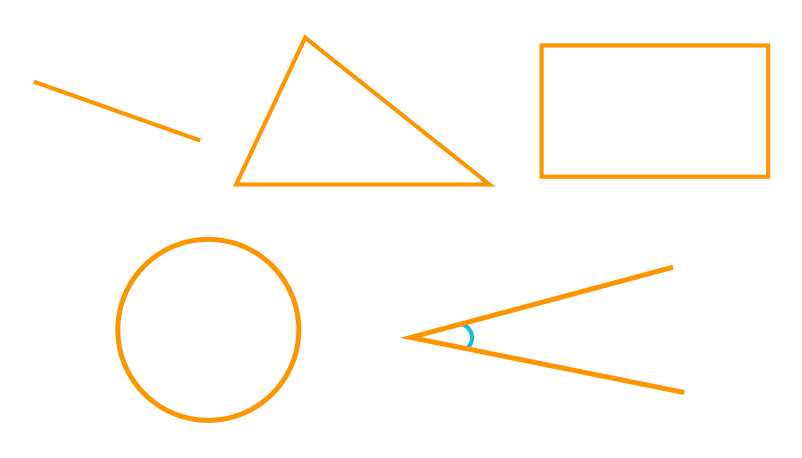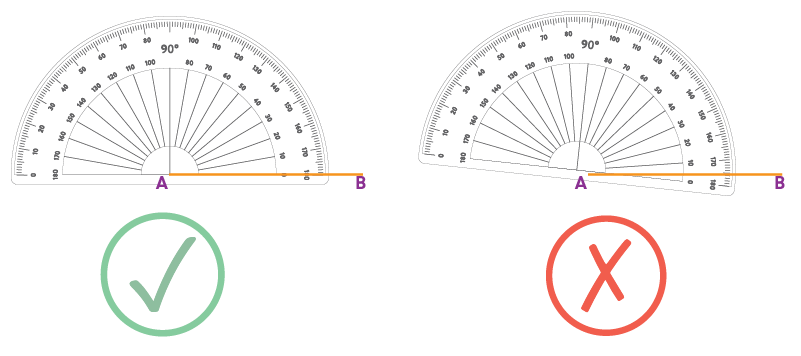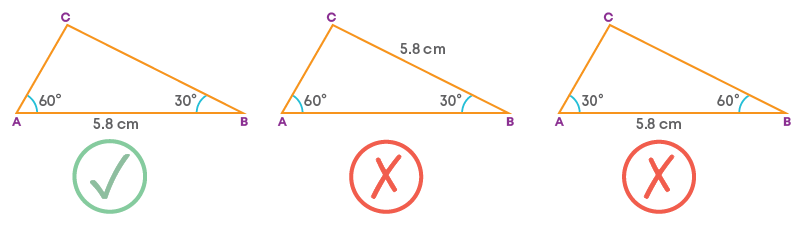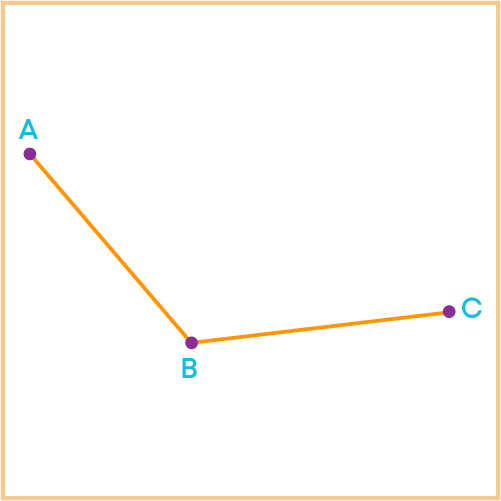## Introduction

In the earlier classes, we have learnt about different geometrical shapes and their properties.Also, we have learnt how to draw line segments of a given length, angles of a given measure, etc. We learn all these constructions under practical geometry.

Practical geometry is a branch of geometry in which the construction of geometrical figures is studied. It involves drawing, measuring, constructing, etc.

Tarun is trying to make a pattern using squares and rectangles. Let us watch this video to know what pattern it is and how it will be made.

In the video above, we saw why we need practical geometry.

#### Concepts

The chapter ‘Practical Geometry’ covers the following concepts:

#### Construction of Parallel Lines

Steps of construction of a line parallel to the given line through a point outside the line:

1. 1. Mark a point outside the given line and join it to a point on the given line.
2. 2.Construct a copy of the angle formed at this point on the line, at the point marked outside the line such that the two angles form a pair of alternate interior angles.
3. 3.One arm of each angle will be common to both. Extend the other arm of the copied angle to get a line.
4. This line will be parallel to the given line as the alternate interior angles are equal.

#### Construction of Triangles

What does SSS criterion of congruence say? It says that two triangles are said to be congruent if three sides of one triangle are equal to the corresponding three sides of the other, i.e., if we know the measures of the three sides of both triangles, we can decide whether the triangles are congruent or not.

Similarly, we have SAS, ASA, AAS and RHS criteria to verify congruence of two triangles.

So, the following sets of measurements decide the congruence of two triangles:

1. 1.Three sides.
2. 2.Two sides and the angle between them.
3. 3.Two angles and the side between them.
4. 4.The hypotenuse and a leg in the case of a right-angled triangle.

So, one of these sets of measurements should be known to construct triangles.

1. 1.Draw a line segment of one of the given lengths as the base of the triangle.
2. 2.Using a compass, keeping one endpoint of the line segment as the centre and with the radius equal to one of the remaining lengths, draw an arc.
3. 3.Keeping the other endpoint of the line segment as the centre and with the radius equal to the remaining length, draw another arc cutting the previous arc.
4. 4.Join the point of intersection of the arcs to the endpoints of the line segment.

This is known as SSS criterion of construction of triangles.

To construct a triangle when the measures of its two sides and the angle between them are given:

1. 1.Draw a line segment of one of the given lengths as the base of the triangle.
2. 2.Using a protractor, at one endpoint of the line segment draw a ray forming the given angle.
3. 3.Using a compass, keeping the same endpoint of the line segment as the centre and with the radius equal to the remaining length, draw an arc cutting the ray.
4. 4.Join the point of intersection of the arc and the ray to the other endpoint of the line segment.

This is known as SAS criterion of construction of triangles.

To construct a triangle when the measures of its two angles and the side between them are given:

1. 1.Draw a line segment of the given length as the base of the triangle.
2. 2.Using a protractor, at one endpoint of the line segment draw a ray forming one of the given angles.
3. 3.At the other endpoint of the line segment draw a ray forming the other given angle.
4. 4.The point of intersection of the rays is one of the vertices of the required triangle.

This is known as ASA criterion of construction of triangles.

To construct a right triangle when the measures of its hypotenuse and a side are given:

1. 1.Draw a line segment of the given length of one side as the base of the triangle.
2. 2.At one endpoint of the line segment draw/construct a ray forming a right angle.
3. 3.Using a compass, with the other endpoint of the line segment as the centre and with the radius equal to the length of the hypotenuse, draw an arc cutting the ray.
4. 4.Join the point of intersection to the point from where the arc is drawn.

This is known as RHS criterion of construction of triangles.

#### Common Errors

The following are topics in which students make common mistakes when dealing with practical geometry:

• 1. Drawing angles
• 2. Drawing rough sketches

#### Drawing Angles

While constructing a triangle with given measures (SAS and ASA), to draw an angle using a protractor, the origin of the protractor should be kept at the proper vertex. Example:

To construct a triangle ABC with AB = 5 cm, ∠A = 60⁰ and ∠B = 48⁰, the angle measuring 60⁰ should be drawn at the vertex A only and the angle measuring 48⁰ should be drawn at the vertex B only.

They should not be interchanged.

Also, while drawing the angles, make sure that the centre of the protractor is coinciding with the proper vertex and the baseline of the protractor is kept along the side of the triangle drawn.#### Drawing Rough Sketches

While drawing rough sketches of triangles before the construction, make sure that you mark the parts of the triangle according to the measures given in the question. Do not interchange them or mark wrong parts with the given measures. Example:

Rough sketch of the triangle ABC with ∠A = 60⁰, ∠B = 30⁰, and the side between them measuring 5.8 cm:#### Conclusion

Geometry helps bring shapes and lines to life by forming meaningful images. Everything around us is constructed and has measurements. Hence, it is important to know how shapes, angles, and lines are constructed.

Practical geometry allows you to construct geometric shapes using simple tools such as protractor, compass, ruler, etc. You may not realise it, but every time you draw something, you make use of such shapes and lines.

So, now can you solve this riddle based on practical geometry?If a line parallel to AB is drawn through C and a line parallel to BC is drawn through A, which of the following figures is obtained?